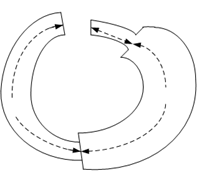## Composite Series Magnetic Circuit Assignment Help

Assignment Help: >> ElectroMagnetism - Composite Series Magnetic Circuit

Composite Series Magnetic Circuit:

A magnetic circuit can consist of different magnetic materials. Now let us assume a composite series magnetic circuit containing three different magnetic materials A, B and C of different permeabilities as well as lengths and air gap as illustrated in Figure. Here the total reluctance shall be the sum of individual reluctances as each path has its own reluctance.Figure: Composite Series Magnetic Circuit

Total reluctance = l/ μ0 μr A

=   (l1/ μ0 μr1 A1)  + (l2/ μ0 μr 2 A2  )  + (l3/ μ0 μr3 A3)  + (la   / μ0 Ag)